Factorial Designs

# Factorial Designs

## A Simple Example

Probably the easiest way to begin understanding factorial designs is by looking at an example. Let’s imagine a design where we have an educational program where we would like to look at a variety of program variations to see which works best. For instance, we would like to vary the amount of time the children receive instruction with one group getting 1 hour of instruction per week and another getting 4 hours per week. And, we’d like to vary the setting with one group getting the instruction in-class (probably pulled off into a corner of the classroom) and the other group being pulled-out of the classroom for instruction in another room. We could think about having four separate groups to do this, but when we are varying the amount of time in instruction, what setting would we use: in-class or pull-out? And, when we were studying setting, what amount of instruction time would we use: 1 hour, 4 hours, or something else?

With factorial designs, we don’t have to compromise when answering these questions. We can have it both ways if we cross each of our two time in instruction conditions with each of our two settings. Let’s begin by doing some defining of terms. In factorial designs, a factor is a major independent variable. In this example we have two factors: time in instruction and setting. A level is a subdivision of a factor. In this example, time in instruction has two levels and setting has two levels. Sometimes we depict a factorial design with a numbering notation. In this example, we can say that we have a `2 x 2` (spoken “two-by-two) factorial design. In this notation, the number of numbers tells you how many factors there are and the number values tell you how many levels. If I said I had a `3 x 4` factorial design, you would know that I had 2 factors and that one factor had 3 levels while the other had 4. Order of the numbers makes no difference and we could just as easily term this a `4 x 3` factorial design. The number of different treatment groups that we have in any factorial design can easily be determined by multiplying through the number notation. For instance, in our example we have `2 x 2 = 4` groups. In our notational example, we would need `3 x 4 = 12` groups.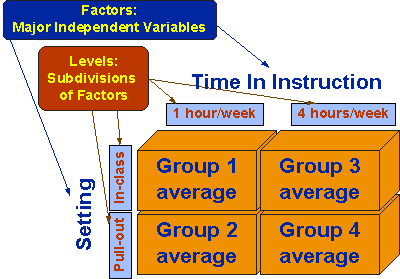We can also depict a factorial design in design notation. Because of the treatment level combinations, it is useful to use subscripts on the treatment (X) symbol. We can see in the figure that there are four groups, one for each combination of levels of factors. It is also immediately apparent that the groups were randomly assigned and that this is a posttest-only design.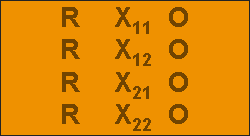Now, let’s look at a variety of different results we might get from this simple `2 x 2` factorial design. Each of the following figures describes a different possible outcome. And each outcome is shown in table form (the `2 x 2` table with the row and column averages) and in graphic form (with each factor taking a turn on the horizontal axis). You should convince yourself that the information in the tables agrees with the information in both of the graphs. You should also convince yourself that the pair of graphs in each figure show the exact same information graphed in two different ways. The lines that are shown in the graphs are technically not necessary – they are used as a visual aid to enable you to easily track where the averages for a single level go across levels of another factor. Keep in mind that the values shown in the tables and graphs are group averages on the outcome variable of interest. In this example, the outcome might be a test of achievement in the subject being taught. We will assume that scores on this test range from `1 to 10` with higher values indicating greater achievement. You should study carefully the outcomes in each figure in order to understand the differences between these cases.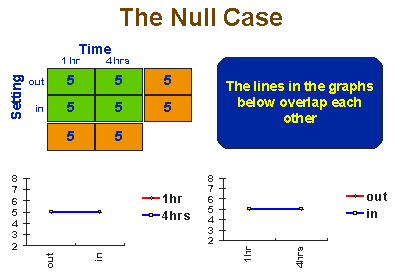## The Null Outcome

Let’s begin by looking at the “null” case. The null case is a situation where the treatments have no effect. This figure assumes that even if we didn’t give the training we could expect that students would score a 5 on average on the outcome test. You can see in this hypothetical case that all four groups score an average of 5 and therefore the row and column averages must be 5. You can’t see the lines for both levels in the graphs because one line falls right on top of the other.

## The Main Effects

A main effect is an outcome that is a consistent difference between levels of a factor. For instance, we would say there’s a main effect for setting if we find a statistical difference between the averages for the in-class and pull-out groups, at all levels of time in instruction. The first figure depicts a main effect of time. For all settings, the 4 hour/week condition worked better than the 1 hour/week one. It is also possible to have a main effect for setting (and none for time).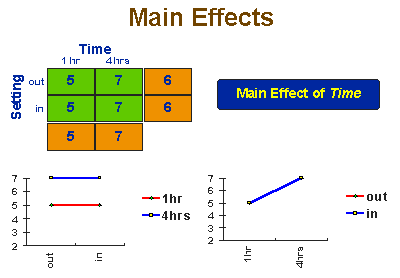In the second main effect graph we see that in-class training was better than pull-out training for all amounts of time.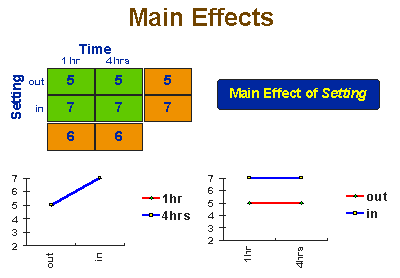Finally, it is possible to have a main effect on both variables simultaneously as depicted in the third main effect figure. In this instance 4 hours/week always works better than 1 hour/week and in-class setting always works better than pull-out.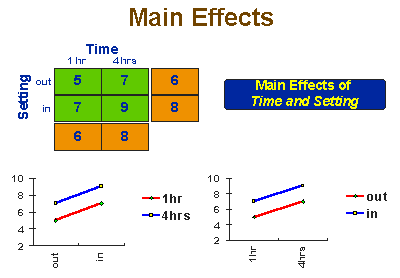## Interaction Effects

If we could only look at main effects, factorial designs would be useful. But, because of the way we combine levels in factorial designs, they also enable us to examine the interaction effects that exist between factors. An interaction effect exists when differences on one factor depend on the level you are on another factor. It’s important to recognize that an interaction is between factors, not levels. We wouldn’t say there’s an interaction between 4 hours/week and in-class treatment. Instead, we would say that there’s an interaction between time and setting, and then we would go on to describe the specific levels involved.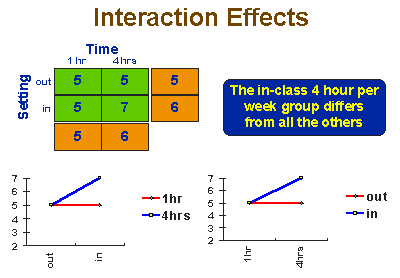How do you know if there is an interaction in a factorial design? There are three ways you can determine there’s an interaction. First, when you run the statistical analysis, the statistical table will report on all main effects and interactions. Second, you know there’s an interaction when can’t talk about effect on one factor without mentioning the other factor. if you can say at the end of our study that time in instruction makes a difference, then you know that you have a main effect and not an interaction (because you did not have to mention the setting factor when describing the results for time). On the other hand, when you have an interaction it is impossible to describe your results accurately without mentioning both factors. Finally, you can always spot an interaction in the graphs of group means – whenever there are lines that are not parallel there is an interaction present! If you check out the main effect graphs above, you will notice that all of the lines within a graph are parallel. In contrast, for all of the interaction graphs, you will see that the lines are not parallel.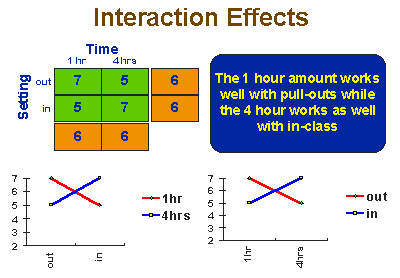In the first interaction effect graph, we see that one combination of levels – 4 hours/week and in-class setting – does better than the other three. In the second interaction we have a more complex “cross-over” interaction. Here, at 1 hour/week the pull-out group does better than the in-class group while at 4 hours/week the reverse is true. Furthermore, the both of these combinations of levels do equally well.

## Summary

Factorial design has several important features. First, it has great flexibility for exploring or enhancing the “signal” (treatment) in our studies. Whenever we are interested in examining treatment variations, factorial designs should be strong candidates as the designs of choice. Second, factorial designs are efficient. Instead of conducting a series of independent studies we are effectively able to combine these studies into one. Finally, factorial designs are the only effective way to examine interaction effects.

So far, we have only looked at a very simple `2 x 2` factorial design structure. You may want to look at some factorial design variations to get a deeper understanding of how they work. You may also want to examine how we approach the statistical analysis of factorial experimental designs.